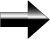Translations Of Graphs

As most math experts know, the secret to solving a complex math problem is to turn it into a simpler problem that you already know how to solve.  This section applies this key concept to graphing complicated functions.  By recognizing the basic graph shapes and understanding the basic rules of translating those graphs, you will be able to sketch or even visualize the graph of almost any function you come across without the help of software, graphing calculators, or tables of values.

We begin with a function f(x), which we know the basic shape of.  For this example, we will use the function f(x) = x2, which is the familiar parabola graph.

For our explanations here, a, b, and c are real numbers.

Horizontal Shift:  f(x + a) shifts the graph to the left by a units.  That also means that if a is negative, it will shift the graph to the right

Horizontal Shrinking or Stretching:  f(c*x) shrinks the graph horizontally by a factor of c (if c > 1).  In other words, all of the x values are divided by c.  Note that this means that when c is less than 1, the graph will be stretched horizontally.

Reflection Over the y-axis:  f(-x) reflects the graph over the y-axis.  By putting a negative in front of the x-values, they all take on the opposite value – this causes the reflection.

Vertical Shift:  f(x) + b shifts the graph up by b units.  If b is negative then it shifts it down b units.  This is because we are adding a number directly to f(x).  This is the easiest way to remember this rule – you’re simply adding a number to the y-value – thus it moves the graph up.

Vertical Shrinking or Stretching:  c*f(x) stretches the graph vertically by a factor of c, if c > 1.  If c < 1, then it shrinks the graph vertically.  The reason for this is simple:  we are multiplying the y- value by c.  So if c > 1, the value of y increases.  If c < 1, the value of y decreases.

Reflection Over the y-axis:  -f(x) reflects the graph over the x-axis.  By putting a negative in front of the y-values, we move it to the other side of the x-axis.  This is what causes the reflection – it is the reflection.  Note that reflections are the same thing as shrinking or stretching, since we are multiplying by a constant.  In the case of reflections, the constant is 1, so it doesn’t stretch or shrink, it just reflects.

Main Algebra Page